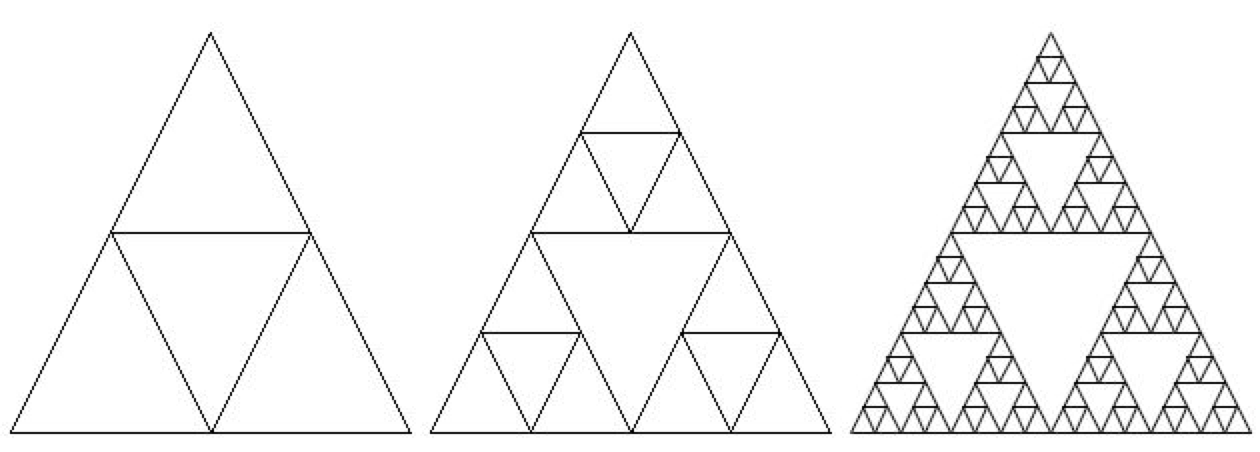# 11.1.1. What is Recursion?¶

Recursion is when a method calls itself. See the example method below.

 ```1 2 3 4 5``` ```public static void neverEnd() { System.out.println("This is the method that never ends!"); neverEnd(); } ```

This method will print out “This is the method that never ends!” and then call itself, which will print out the message again, and then call itself, and so on. This is called infinite recursion, which is a recursion that never ends. Of course, this particular method is not very useful.Check your Understanding

# 11.1.2. Why use Recursion?¶

Recursion is most useful when it is used to solve problems where the structure of the problem repeats. For example, what if you wanted to find out how much space a folder on your computers uses? You could add up the sizes of all the files in that folder, but folders can also contain subfolders. So you will have to repeat the procedure (method) for each subfolder. Each subfolder can also contain subfolders.

Recursion can also be used to create fractals. A simple example is Sierpinski’s triangle in which you subdivide a triangle into 4 new triangles as shown below. You can then do the some procedure with each new triangle except the center one.Figure 1: A sequence of Sierpinski’s triangles

Recursion can also be used to traverse String, array, and ArrayList objects, much like a loop. In fact, any recursive solution could be written with iteration (loops) instead.

# 11.1.3. Factorial Method¶

The following video is also on YouTube at https://youtu.be/V2S_8E_ubBY. It introduces the concept of recursion and tracing recursion with the factorial method.

See the method factorial below that calculates the factorial of a number. The factorial of a number is defined as 1 for 0 and n * factorial (n-1) for any other number.

 ```1 2 3 4 5 6 7``` ```public static int factorial(int n) { if (n == 0) return 1; else return n * factorial(n-1); } ```Check your understanding

Run the code below to test the factorial method. What’s the factorial of 6? Add another test to print out the factorial of 6. What’s the factorial of 1? Add another test to print out the factorial of 1.

# 11.1.4. Base Case¶

Every recursive method must have at least one base case which halts the recursion. This is usually an if statement that causes the recursion to stop by just giving an answer without needing a recursive method call. You could also think of it as the simplest case where you can give the answer right away. The factorial method has a way to stop the recursion (not call itself). It stops when n is equal to 0, since it just returns 1. This is the base case.

Note

The thing that stops a recursive method from calling itself is called the base case. A method can have more than one base case (way to stop the recursion).Check your understanding

Continue to the next page for Day 2 of the Recursion lesson.